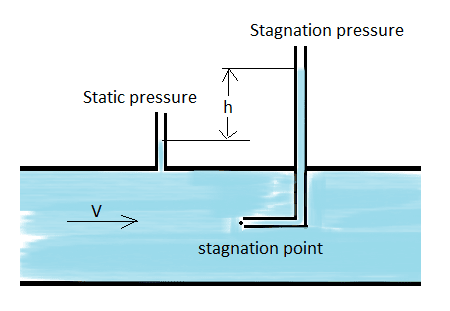What Is Pitot tube? Construction, Working Principle of Pitot tube | Mecholic -->

# What Is Pitot tube? Construction, Working Principle of Pitot tubePitot tube for velocity measurement and flow measurement

## Construction of pitot tube

It is basically a fluid velocity measuring instrument that can also be used for flow measurement of liquids and gases. It consists of two hollow tubes that sense pressure at different places within the pipe. This hollow tubes can be mounted separately in a pipe or installing together in one casing as a single device. One tube measures the stagnation or impact pressure and another tube measures only static pressure usually at the wall of the pipe.

## Working principle of Pitot tube

When a solid body is kept centrally, and stationery in a pipeline with flowing fluid, the velocity of the fluid starts reducing (at the same time the pressure fluid increases due to the conversion of kinetic energy into pressure energy) due to the presence of the body. At directly in front of the solid body, the velocity becomes zero. This point is known as the stagnation point.

The fluid flow can be measured by measuring the differences between the pressure at the normal flow line (static pressure) and the stagnation point (stagnation pressure).

- Applications, advantages and limitations of Pitot tubes
- Combined pitot tube (Prandtl Tube) and Double tip pitot tube
Difference between pitot tube and Pitot-static tube

## Pitot tube velocity equation

The equation of velocity is derived by applying Bernoulli’s principle; the final equation is given below
Velocity,g = acceleration due to gravity
Actual velocity,Cv = Coefficient velocity of pitot tube
• The operating principle of pitot tube: conversion of kinetic head into pressure head